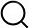# Also in the Article

Description of the CG polymer model
This protocol is extracted from research article:
Energy renormalization for coarse-graining polymers having different segmental structures

Procedure

To coarse-grain the AA BPA-PC model, we applied a four-beads-per-monomer mapping scheme, with three different CG bead types A, B, and C, representing phenylene, isopropylidene, and carbonate groups, respectively (Fig. 1A). Figure 1B shows a snapshot of the CG model of one PC chain with a chain length of N = 10 (i.e., number of monomers per chain) overlaid with the atomistic model. A similar mapping scheme for coarse-graining PC has also been adopted in previous works (2022). The bonded interactions of the CG model, including bonds, angles, and dihedrals, were derived by matching their probability distributions of the AA model using the IBM. The detailed derivation and description of the coarse-graining procedure for the bonded interactions are shown in the Supplementary Materials.

The non-bonded interactions between the CG beads are represented by the standard 12-6 LJ potential: $U(r)=4ε[(σr)12−(σr)6]$, where σ governs the effective van der Waals radius and marks the distance where U is 0 and ε is the depth of the potential, a parameter associated with the cohesive interaction strength of the material. The cutoff distance of the LJ potential is 1.5 nm. To achieve the temperature transferability, we introduced temperature-dependent renormalization factors, α(T)and β(T), to rescale ε [i.e., ε(T) = α(T0] and σ [i.e., σ(T) = β(T0] for each CG bead type, respectively, where ε0 and σ0 are constants that can be taken as initial estimates of ε and σ from the RDF measurement using the IBM. On the basis of our previous work, β(T) was obtainable by preserving the density of the AA system. Here, we focused more on deriving α(T) for the non-bonded cohesive interactions of the CG model since these interactions play a critical role in the dynamic and thermomechanical properties of GF polymers (46).

Note: The content above has been extracted from a research article, so it may not display correctly.

Q&A